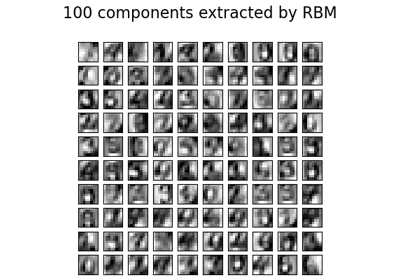# sklearn.neural_network.BernoulliRBM¶

class sklearn.neural_network.BernoulliRBM(n_components=256, *, learning_rate=0.1, batch_size=10, n_iter=10, verbose=0, random_state=None)[source]

Bernoulli Restricted Boltzmann Machine (RBM).

A Restricted Boltzmann Machine with binary visible units and binary hidden units. Parameters are estimated using Stochastic Maximum Likelihood (SML), also known as Persistent Contrastive Divergence (PCD) .

The time complexity of this implementation is O(d ** 2) assuming d ~ n_features ~ n_components.

Read more in the User Guide.

Parameters:
n_componentsint, default=256

Number of binary hidden units.

learning_ratefloat, default=0.1

The learning rate for weight updates. It is highly recommended to tune this hyper-parameter. Reasonable values are in the 10**[0., -3.] range.

batch_sizeint, default=10

Number of examples per minibatch.

n_iterint, default=10

Number of iterations/sweeps over the training dataset to perform during training.

verboseint, default=0

The verbosity level. The default, zero, means silent mode. Range of values is [0, inf].

random_stateint, RandomState instance or None, default=None

Determines random number generation for:

• Gibbs sampling from visible and hidden layers.

• Initializing components, sampling from layers during fit.

• Corrupting the data when scoring samples.

Pass an int for reproducible results across multiple function calls. See Glossary.

Attributes:
intercept_hidden_array-like of shape (n_components,)

Biases of the hidden units.

intercept_visible_array-like of shape (n_features,)

Biases of the visible units.

components_array-like of shape (n_components, n_features)

Weight matrix, where n_features is the number of visible units and n_components is the number of hidden units.

h_samples_array-like of shape (batch_size, n_components)

Hidden Activation sampled from the model distribution, where batch_size is the number of examples per minibatch and n_components is the number of hidden units.

n_features_in_int

Number of features seen during fit.

New in version 0.24.

feature_names_in_ndarray of shape (n_features_in_,)

Names of features seen during fit. Defined only when X has feature names that are all strings.

New in version 1.0.

sklearn.neural_network.MLPRegressor

Multi-layer Perceptron regressor.

sklearn.neural_network.MLPClassifier

Multi-layer Perceptron classifier.

sklearn.decomposition.PCA

An unsupervised linear dimensionality reduction model.

References

 Hinton, G. E., Osindero, S. and Teh, Y. A fast learning algorithm for

deep belief nets. Neural Computation 18, pp 1527-1554. https://www.cs.toronto.edu/~hinton/absps/fastnc.pdf

 Tieleman, T. Training Restricted Boltzmann Machines using

Approximations to the Likelihood Gradient. International Conference on Machine Learning (ICML) 2008

Examples

>>> import numpy as np
>>> from sklearn.neural_network import BernoulliRBM
>>> X = np.array([[0, 0, 0], [0, 1, 1], [1, 0, 1], [1, 1, 1]])
>>> model = BernoulliRBM(n_components=2)
>>> model.fit(X)
BernoulliRBM(n_components=2)


Methods

 fit(X[, y]) Fit the model to the data X. fit_transform(X[, y]) Fit to data, then transform it. get_feature_names_out([input_features]) Get output feature names for transformation. Get metadata routing of this object. get_params([deep]) Get parameters for this estimator. Perform one Gibbs sampling step. partial_fit(X[, y]) Fit the model to the partial segment of the data X. Compute the pseudo-likelihood of X. set_output(*[, transform]) Set output container. set_params(**params) Set the parameters of this estimator. Compute the hidden layer activation probabilities, P(h=1|v=X).
fit(X, y=None)[source]

Fit the model to the data X.

Parameters:
X{array-like, sparse matrix} of shape (n_samples, n_features)

Training data.

yarray-like of shape (n_samples,) or (n_samples, n_outputs), default=None

Target values (None for unsupervised transformations).

Returns:
selfBernoulliRBM

The fitted model.

fit_transform(X, y=None, **fit_params)[source]

Fit to data, then transform it.

Fits transformer to X and y with optional parameters fit_params and returns a transformed version of X.

Parameters:
Xarray-like of shape (n_samples, n_features)

Input samples.

yarray-like of shape (n_samples,) or (n_samples, n_outputs), default=None

Target values (None for unsupervised transformations).

**fit_paramsdict

Returns:
X_newndarray array of shape (n_samples, n_features_new)

Transformed array.

get_feature_names_out(input_features=None)[source]

Get output feature names for transformation.

The feature names out will prefixed by the lowercased class name. For example, if the transformer outputs 3 features, then the feature names out are: ["class_name0", "class_name1", "class_name2"].

Parameters:
input_featuresarray-like of str or None, default=None

Only used to validate feature names with the names seen in fit.

Returns:
feature_names_outndarray of str objects

Transformed feature names.

Get metadata routing of this object.

Please check User Guide on how the routing mechanism works.

Returns:

A MetadataRequest encapsulating routing information.

get_params(deep=True)[source]

Get parameters for this estimator.

Parameters:
deepbool, default=True

If True, will return the parameters for this estimator and contained subobjects that are estimators.

Returns:
paramsdict

Parameter names mapped to their values.

gibbs(v)[source]

Perform one Gibbs sampling step.

Parameters:
vndarray of shape (n_samples, n_features)

Values of the visible layer to start from.

Returns:
v_newndarray of shape (n_samples, n_features)

Values of the visible layer after one Gibbs step.

partial_fit(X, y=None)[source]

Fit the model to the partial segment of the data X.

Parameters:
Xndarray of shape (n_samples, n_features)

Training data.

yarray-like of shape (n_samples,) or (n_samples, n_outputs), default=None

Target values (None for unsupervised transformations).

Returns:
selfBernoulliRBM

The fitted model.

score_samples(X)[source]

Compute the pseudo-likelihood of X.

Parameters:
X{array-like, sparse matrix} of shape (n_samples, n_features)

Values of the visible layer. Must be all-boolean (not checked).

Returns:
pseudo_likelihoodndarray of shape (n_samples,)

Value of the pseudo-likelihood (proxy for likelihood).

Notes

This method is not deterministic: it computes a quantity called the free energy on X, then on a randomly corrupted version of X, and returns the log of the logistic function of the difference.

set_output(*, transform=None)[source]

Set output container.

See Introducing the set_output API for an example on how to use the API.

Parameters:
transform{“default”, “pandas”}, default=None

Configure output of transform and fit_transform.

• "default": Default output format of a transformer

• "pandas": DataFrame output

• None: Transform configuration is unchanged

Returns:
selfestimator instance

Estimator instance.

set_params(**params)[source]

Set the parameters of this estimator.

The method works on simple estimators as well as on nested objects (such as Pipeline). The latter have parameters of the form <component>__<parameter> so that it’s possible to update each component of a nested object.

Parameters:
**paramsdict

Estimator parameters.

Returns:
selfestimator instance

Estimator instance.

transform(X)[source]

Compute the hidden layer activation probabilities, P(h=1|v=X).

Parameters:
X{array-like, sparse matrix} of shape (n_samples, n_features)

The data to be transformed.

Returns:
hndarray of shape (n_samples, n_components)

Latent representations of the data.

## Examples using sklearn.neural_network.BernoulliRBM¶Restricted Boltzmann Machine features for digit classification

Restricted Boltzmann Machine features for digit classification